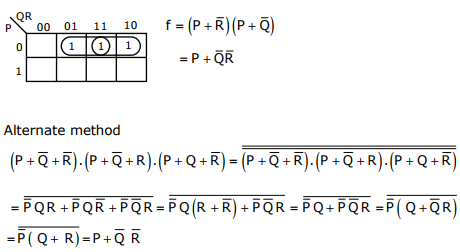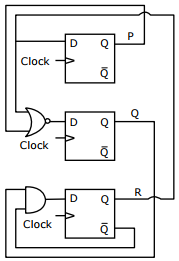# Digital Logic - Online Test

Q1. What is the minimum number of gates required to implement the Boolean function (AB + C) if we have to use only 2-input NOR gates?
Explaination / Solution:
No Explaination.

Q2. The minterm expansion of fExplaination / Solution:
No Explaination.

Q3. P is a 16-bit signed integer. The 2’s complement representation of P is (F87B)16. The 2’s complement representation of 8*P is
Explaination / Solution:
No Explaination.

Q4.
The Boolean expression for the output f of the multiplexer shown below isExplaination / Solution:
No Explaination.

Q5. The simplified SOP (Sum of Product) form of the Boolean expressionis
Explaination / Solution:Q6. Which one of the following circuits is NOT equivalent to a 2-input XNOR (exclusive NOR) gate?
Explaination / Solution:

All options except option ‘D’ gives EX-NOR gates

Q7. The minimum number of D flip-flops needed to design a mod-258 counter is
Explaination / Solution:

2≥ 258 ⇒ n = 9

Q8. Consider the following circuit involving three D-type flip-flops used in a certain type of counter configuration.If all the flip-flops were reset to 0 at power on, what is the total number of distinct outputs (states) represented by PQR generated by the counter?
Explaination / Solution:So Total number of distinct outputs is 4

Q9. Consider the following circuit involving three D-type flip-flops used in a certain type of counter configuration.If at some instance prior to the occurrence of the clock edge, P. Q and R have a value 0, 1 and 0 respectively, what shall be the value of PQR after the clock edge?
Explaination / Solution:

From the Table Shown in the explanation of question 50, if first state is 010 next State is 011

Q10. The decimal value 0.5 in IEEE single precision floating point representation has
Explaination / Solution:

(0.5)10 =  (1.0)2 × 2-1
So, exponent = -1 and fraction is 000 - - - 000